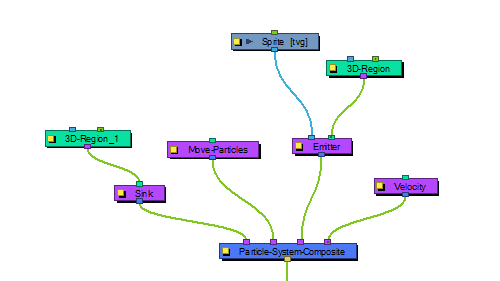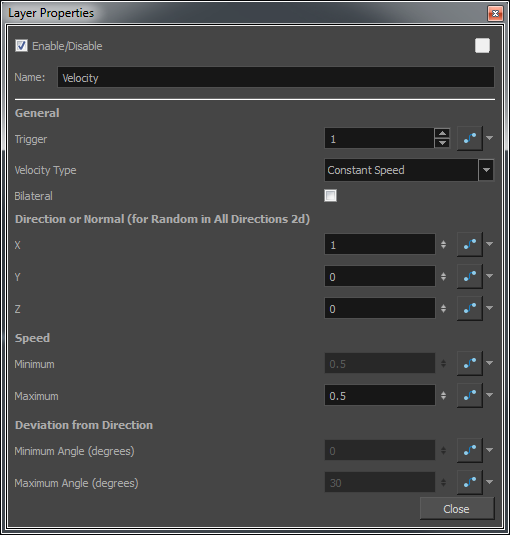# Velocity

The Velocity effect defines the initial velocity of the particles that are emitted in the particle system. You can select this node and select View > Show > Control to display the manipulation handles to help you set the parameters of this node. Use your Transform tool to modify the manipulation handles in the Camera or Perspective view.### Velocity Properties

Parameter Description
General

Trigger: Is an on/off switch using binary code, where 1 = on, while 0 = off.

Velocity Type

 • Use Input Shape: If you plug a Region into the in-port of the Velocity node, it will take the shape of this region to determine the initial velocity of the particles. They will take a velocity that is perpendicular to the surface of the shape.
 • Constant Speed: Sets a constant speed for the initial velocity of the particles.
 • Interpolated Speed: Each particle is assigned an initial speed that is somewhere between the two values set for Interpolated Speed.
 • Random in All Directions 2D: All particles will be assigned a random velocity in any direction on a 2D plane.
 • Random in All Directions 3D: All particles will be assigned a random velocity in any direction.
 • Random in Cone: Particles will be assigned an initial velocity anywhere in the shape of a cone.
 • Random in 2D-Pie: Particles will be assigned an initial velocity anywhere in the shape of a pie slice on a 2D plane.

Bilateral: Generates positive and negative velocities in a given direction. For example, you can generate particles with cone-shaped velocity, (e.g. a bazooka firing) going only in the forward direction, but you can also generate velocities in the opposite direction.

Direction of Normal (for Random in all Directions 2D) Lets you input values for the perpendicular direction for the Random property in All Directions 2D.

X: Sets a value between 0 and 1 to determine how much influence the X-axis has over the direction of the normal. For example, if the X value is set to 1, and Y and Z are set to 0, then the perpendicular (normal) will be going in the X direction. If X is set to 1, and Y is also set to 1, then the axis will be 45 degrees, halfway between X and Y. Feel free to use the Manipulation Handles for the velocity node by selecting the Velocity node and then selecting View > Show > Control. This will allow you to use the Transform tool in the Camera View or the Perspective View to set the appropriate values.

Y: Set a value between 0 and 1 to determine how much influence the Y-axis has over the direction of the normal.

Z: Set a value between 0 and 1 to determine how much influence the Z-axis has over the direction of the normal.

Speed

Minimum: There are some speeds that are constant, and some that are a range of values between a minimum and maximum. Set the minimum speed here.

Maximum: Set the maximum speed for interpolated speeds.

Deviation from Direction

Minimum Angle: This is used for Cone and Pie shapes. Put a non-zero value here if you want there to be a hole in the middle of your cone or pie.

Maximum Angle: Set the maximum angle for your cone or pie here.